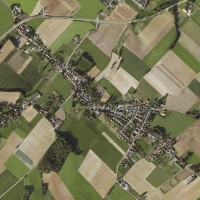Welcome to Bingelder.nl Photography - ConcertsHome   - Home Mardi Gras Indians   - MGI Calendar Photography Hobby Links Recent changes Contact Hi Guys, welcome to the all-new Bingelder.nl   Concert photography. For other concert photography click here. Record Store Day, 21 april 2018, Music Machine, Sittard (NL)Music Machine RSD'18: Blo Ya Top, 21 april 2018, Music Machine, Sittard (NL)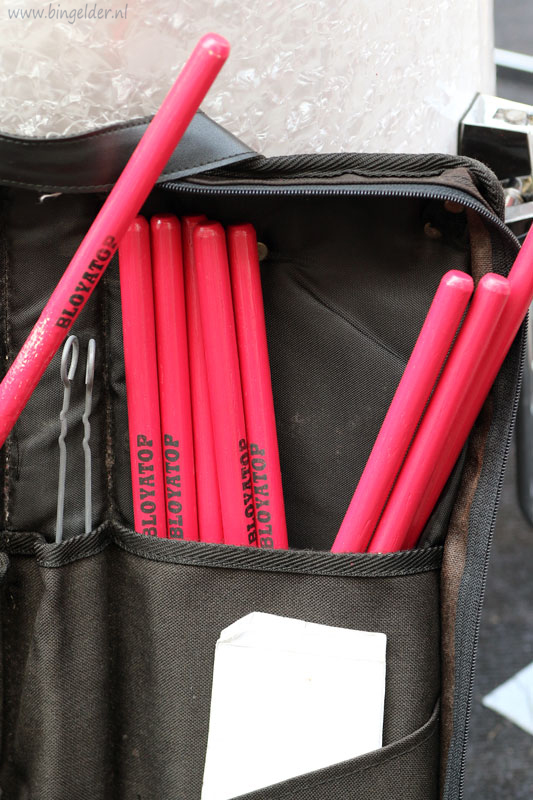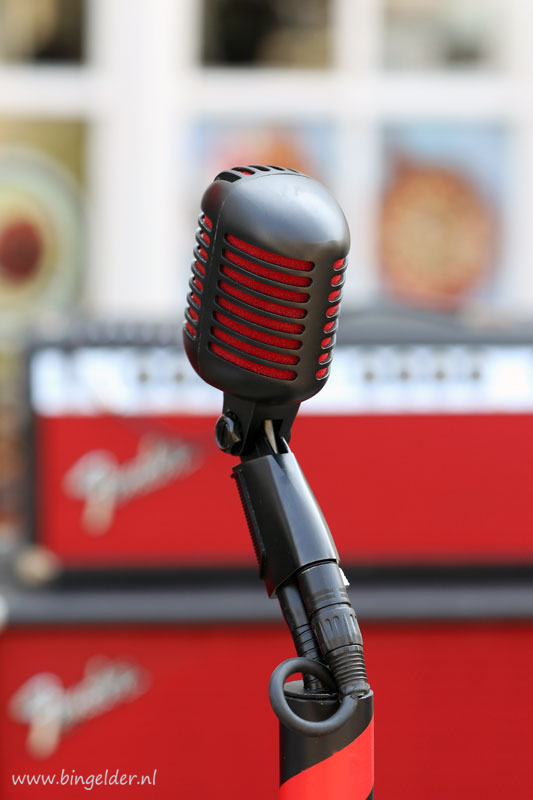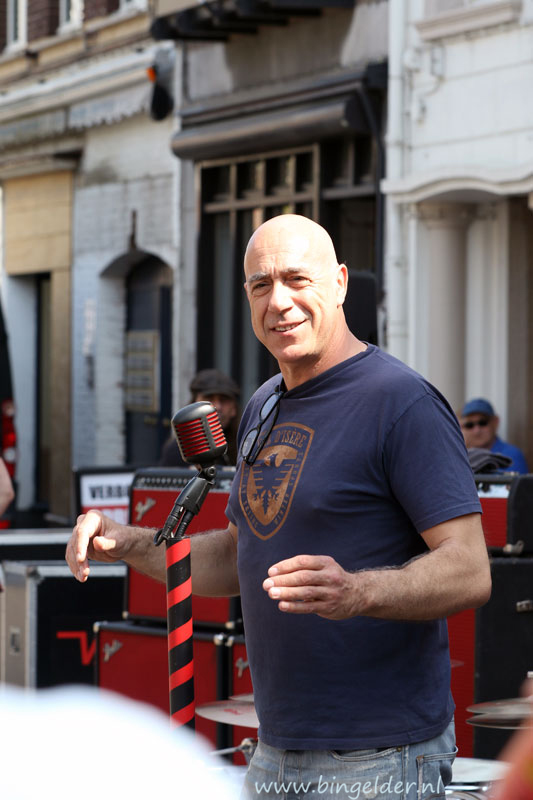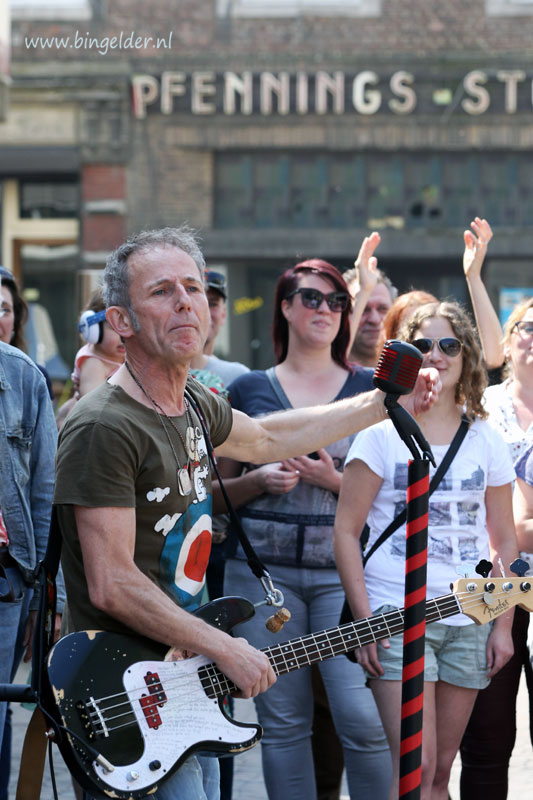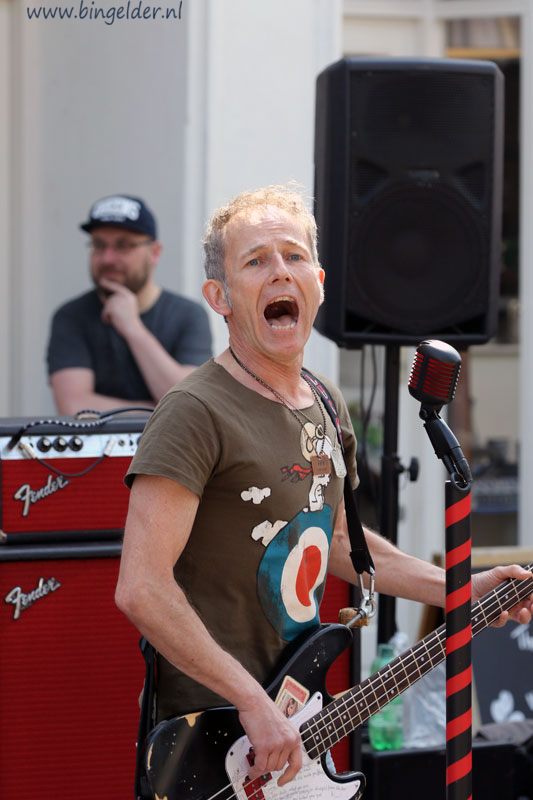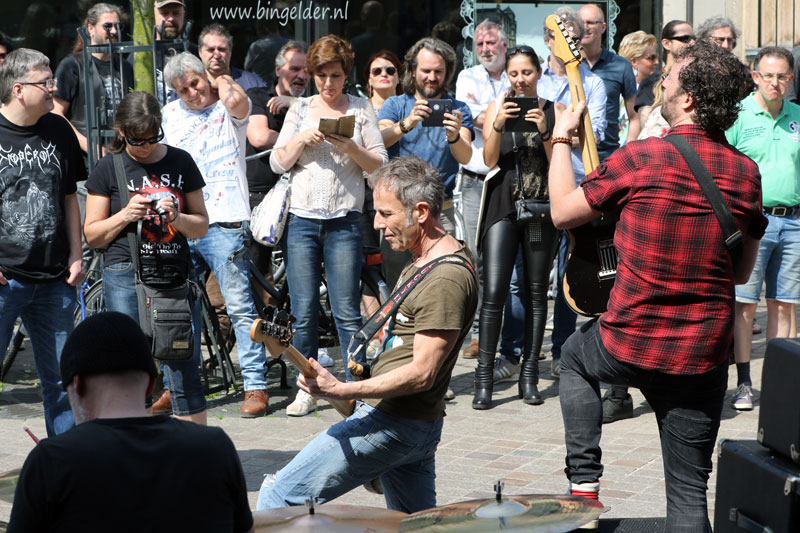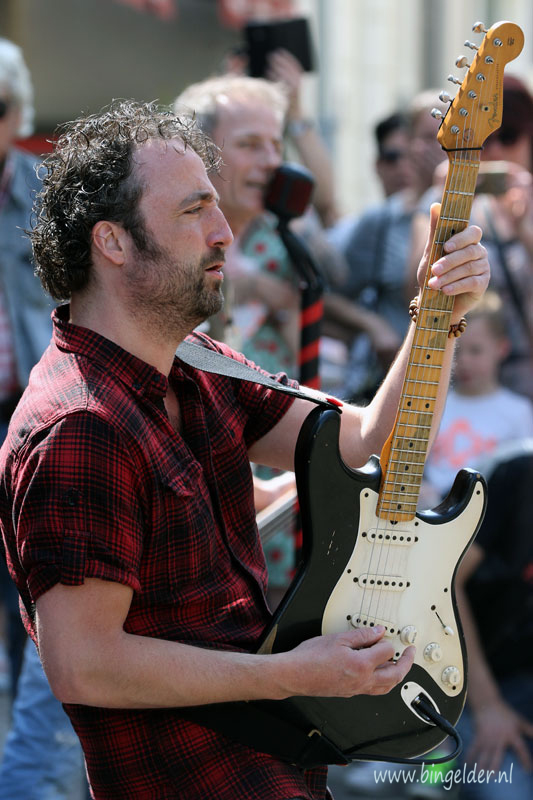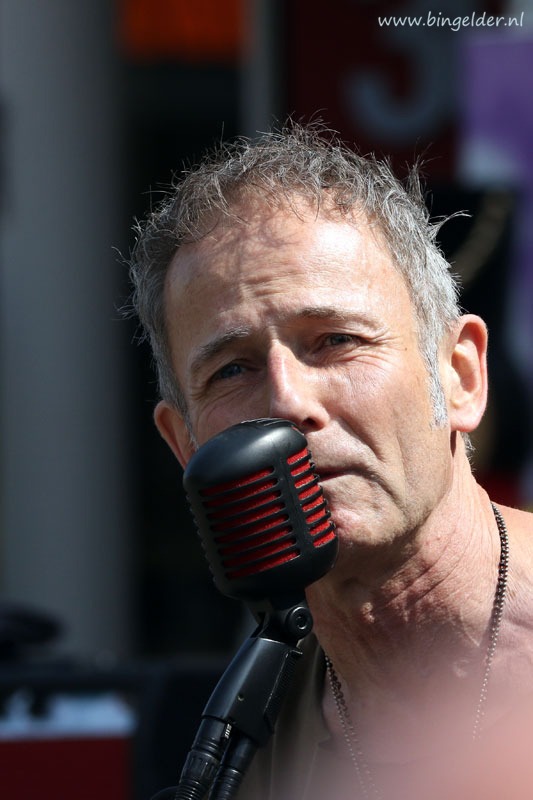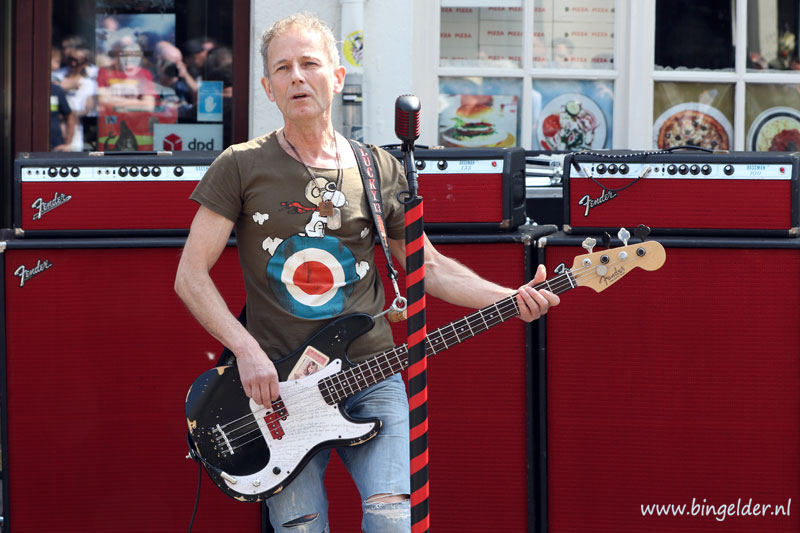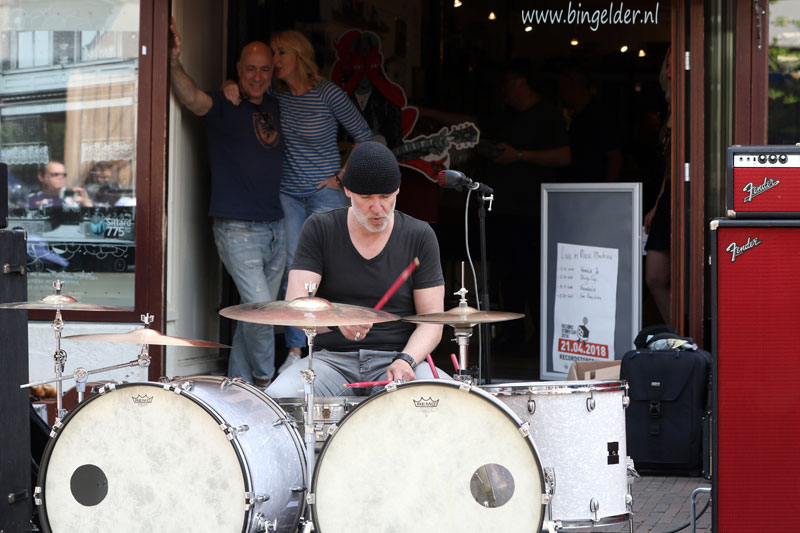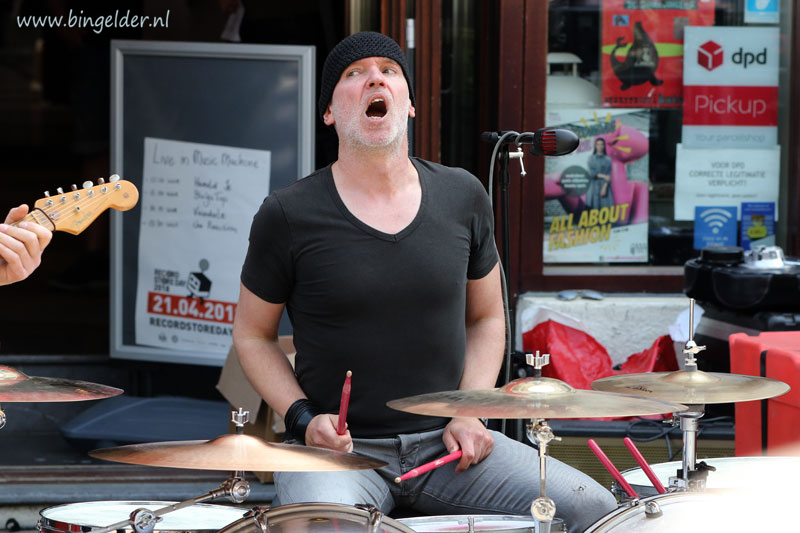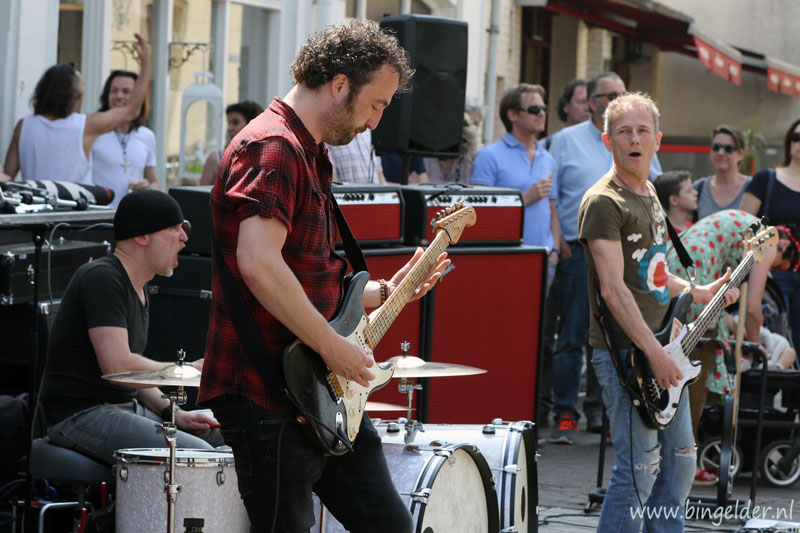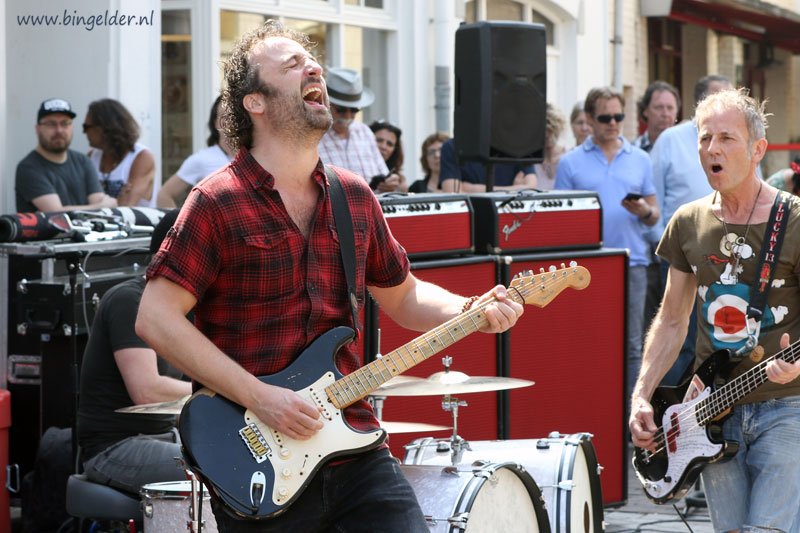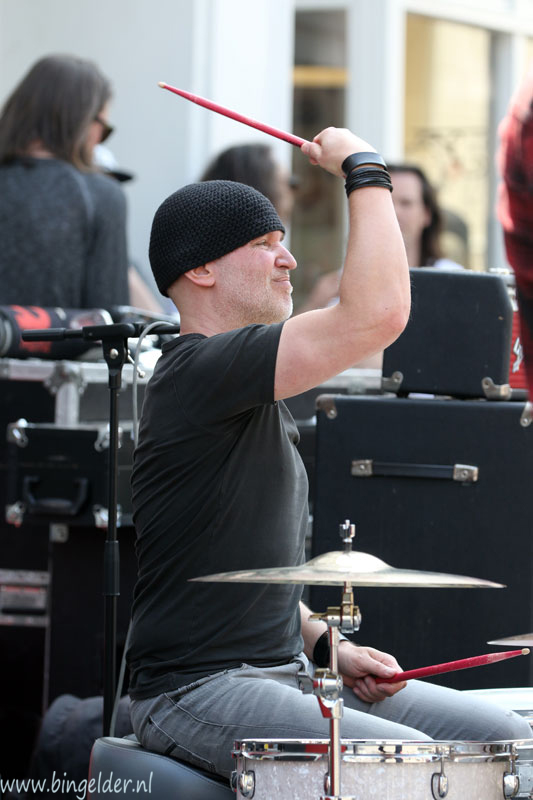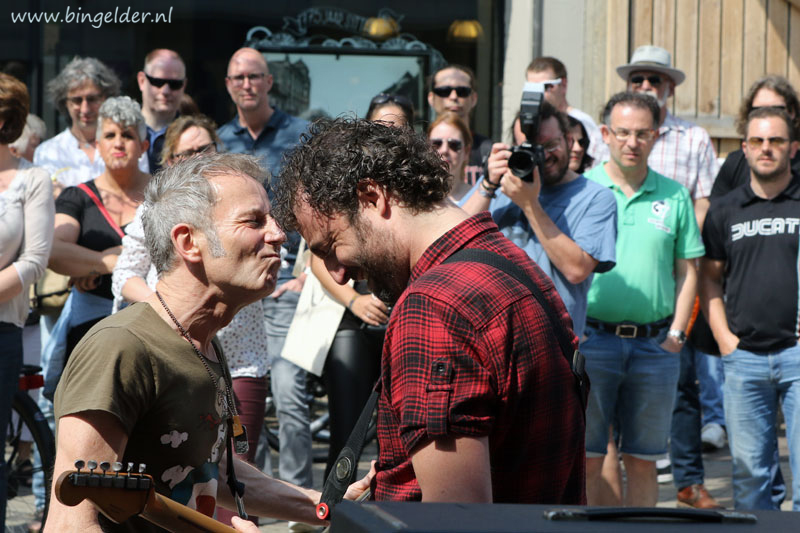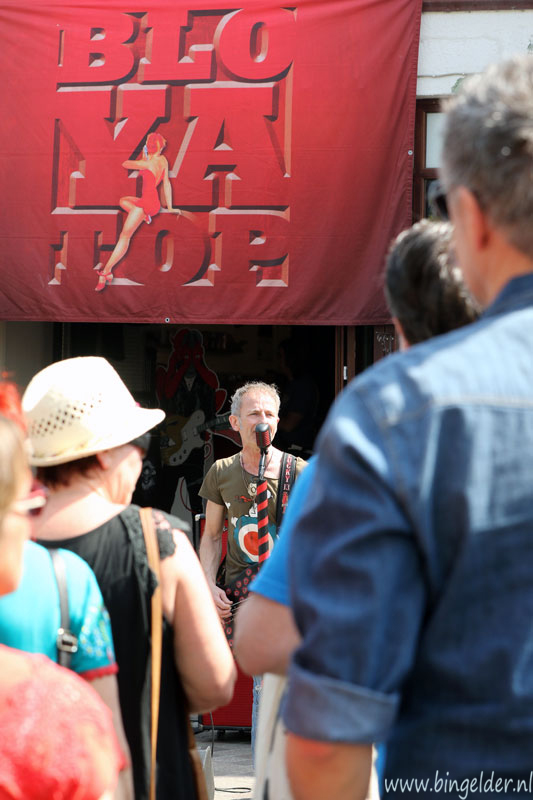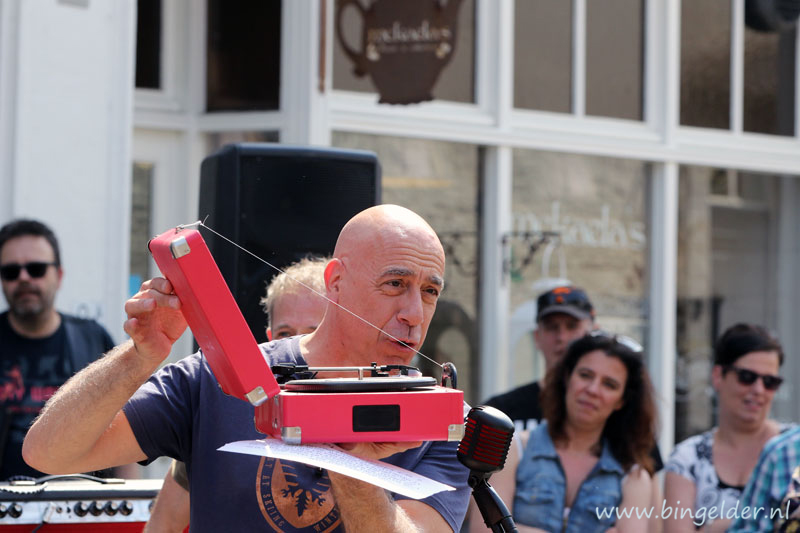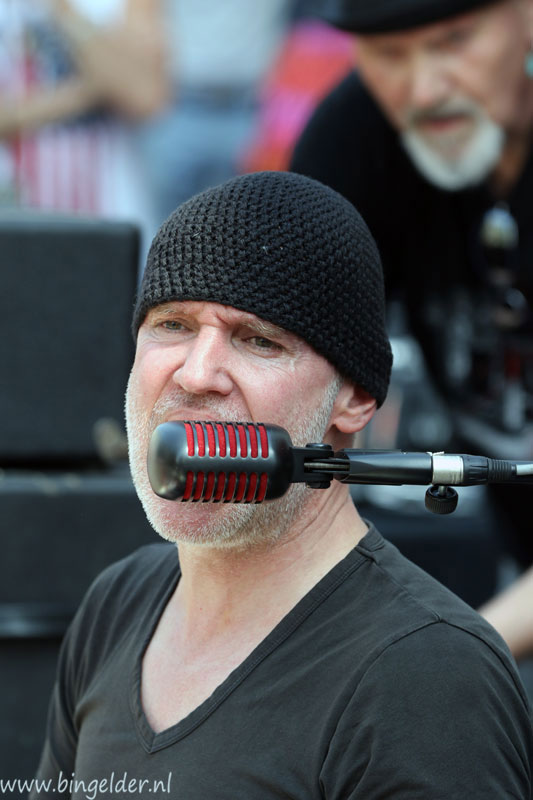RSD'18: Vandale, 21 april 2018, Music Machine, Sittard (NL)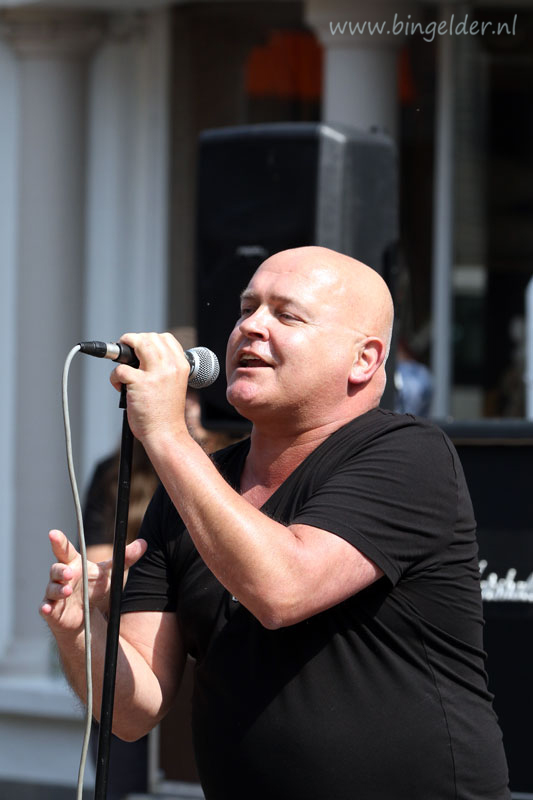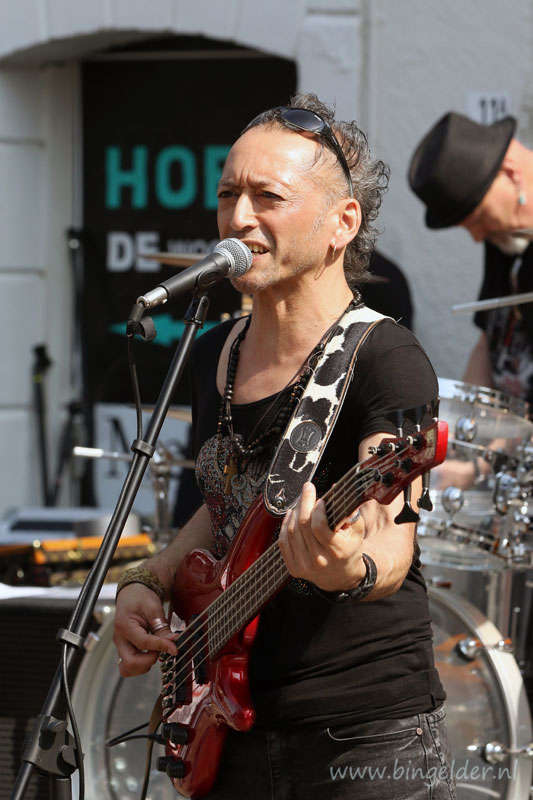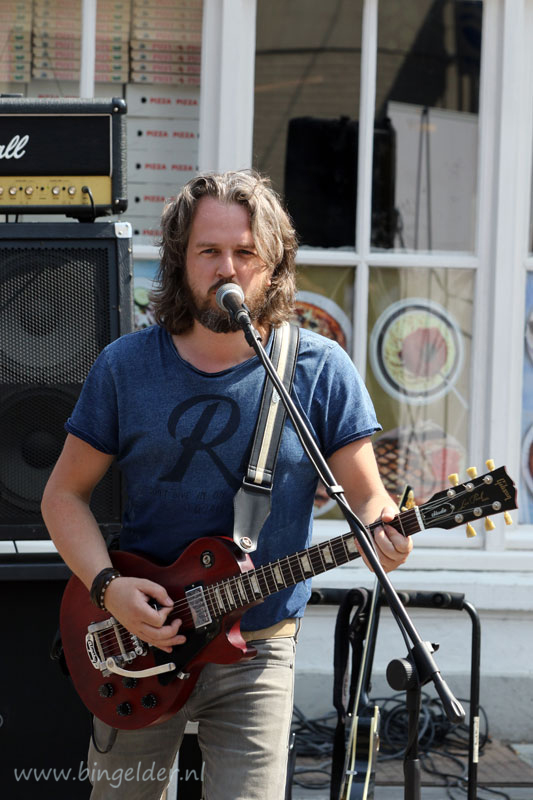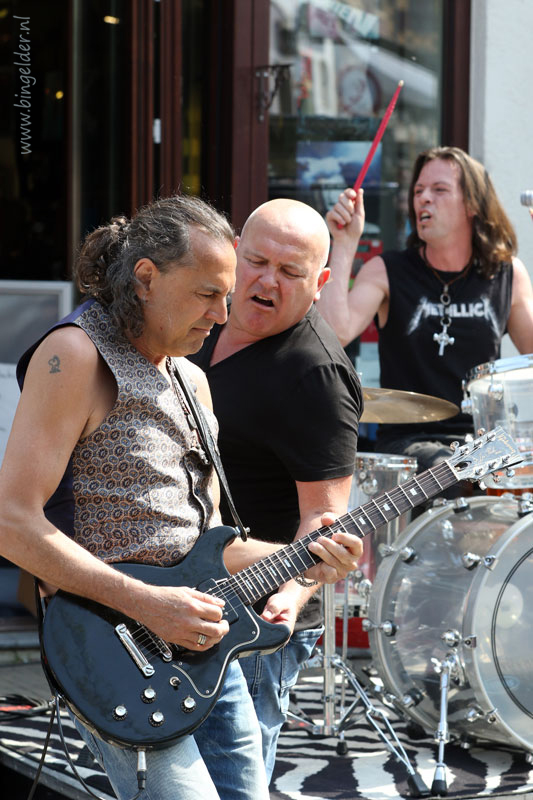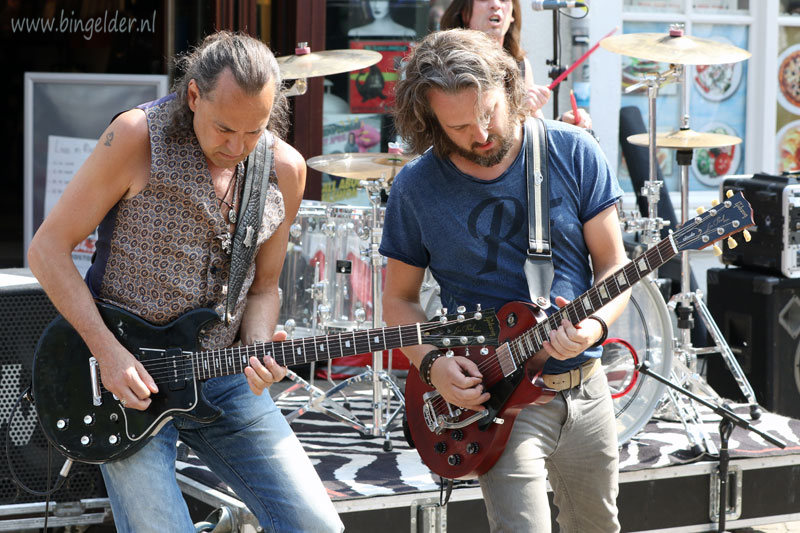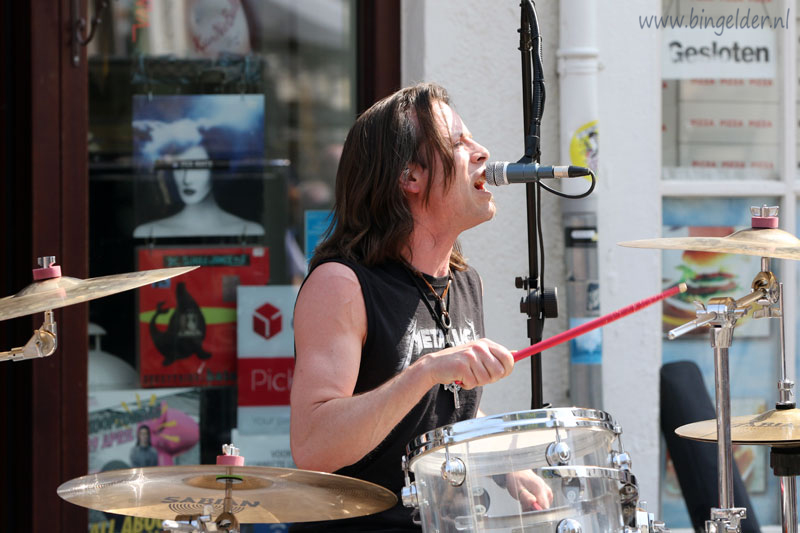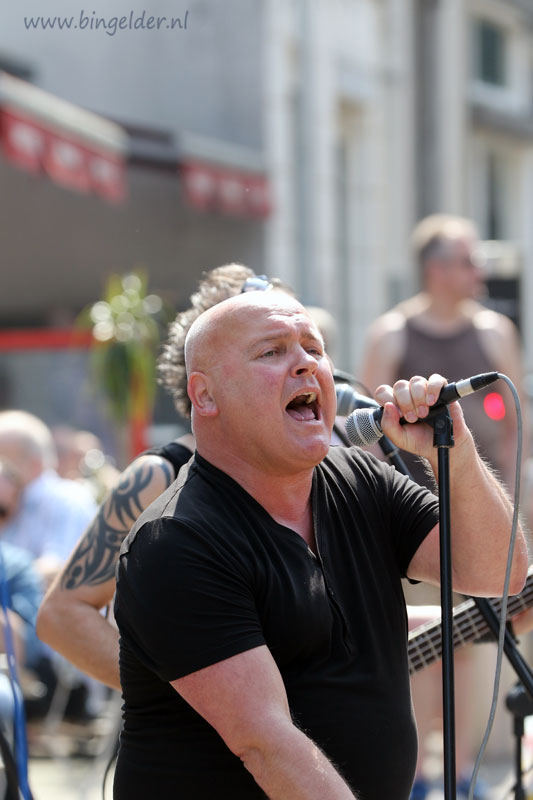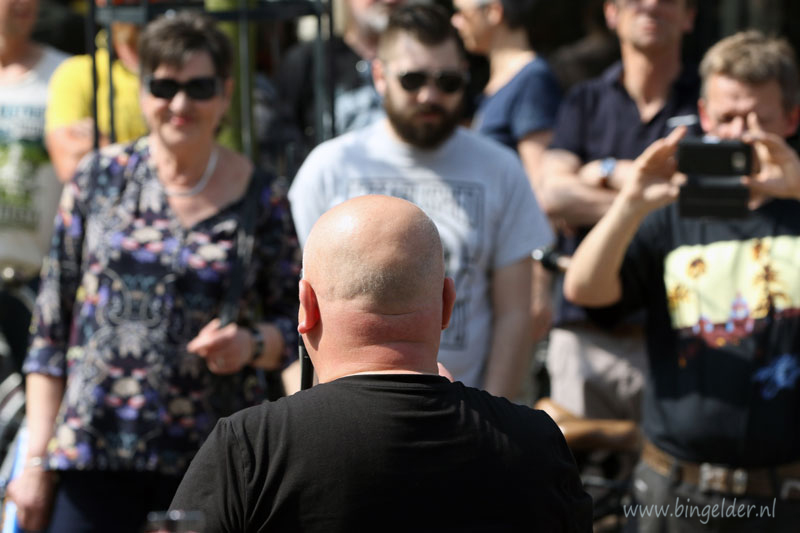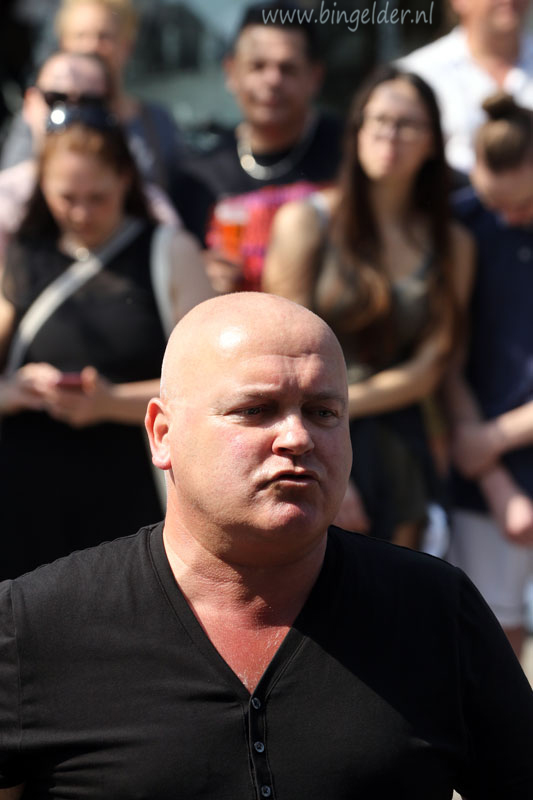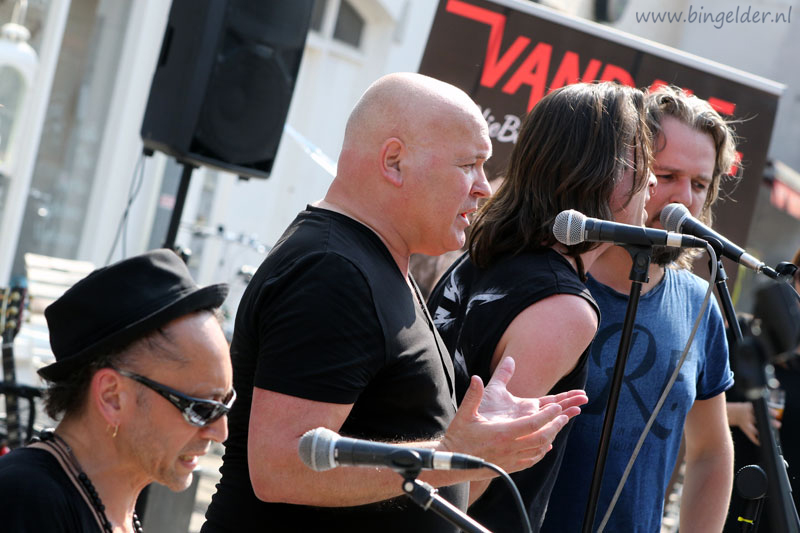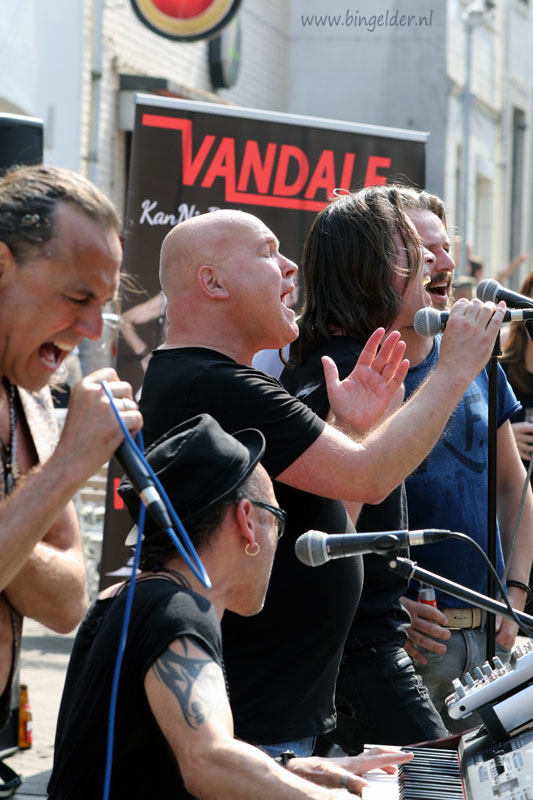RSD'18: The Reaction, 21 april 2018, Music Machine, Sittard (NL)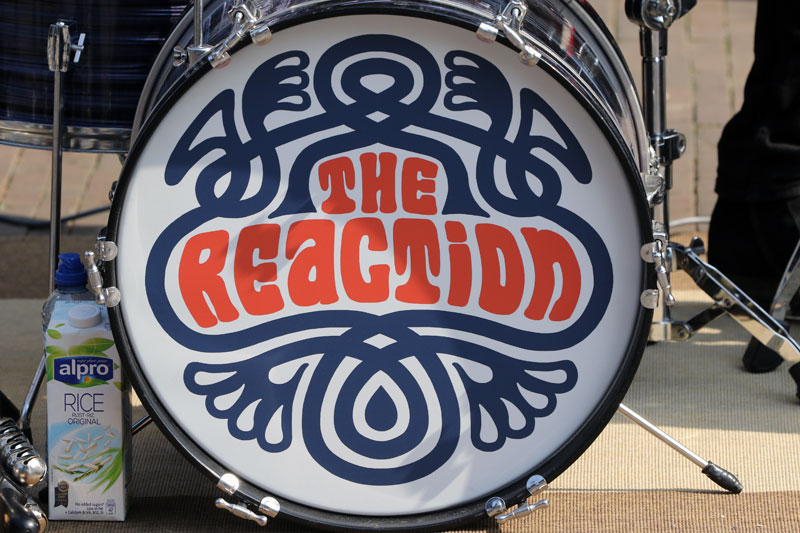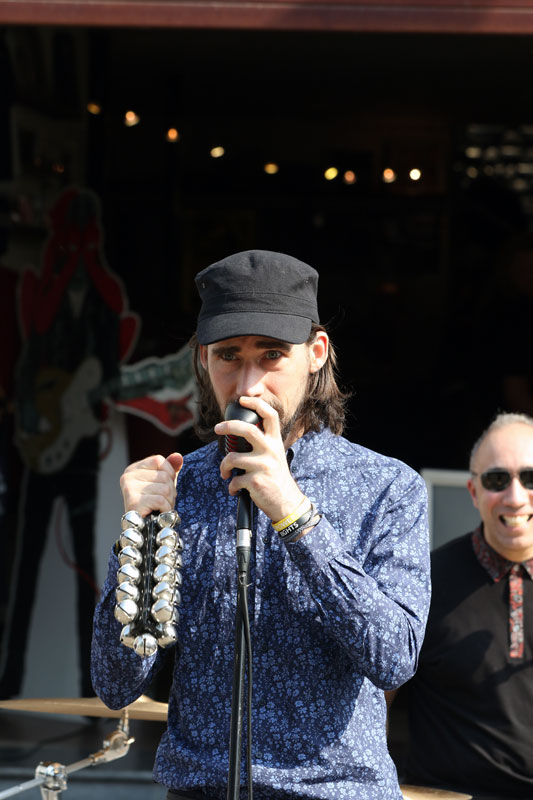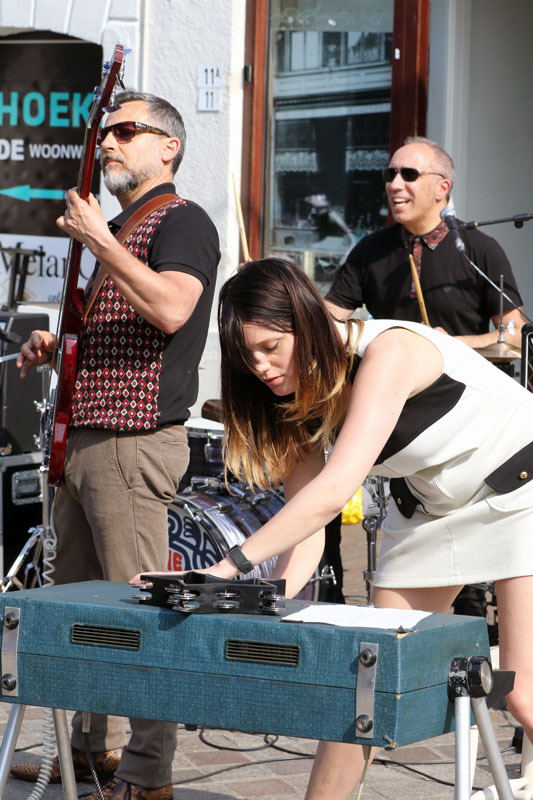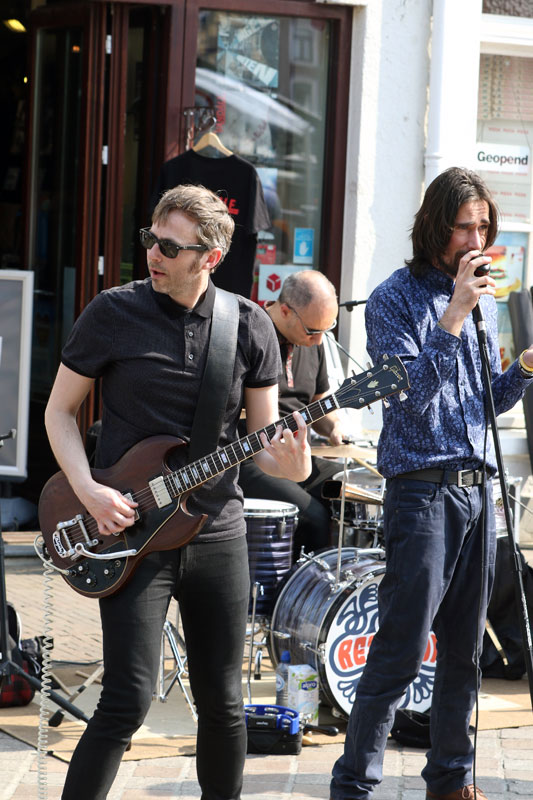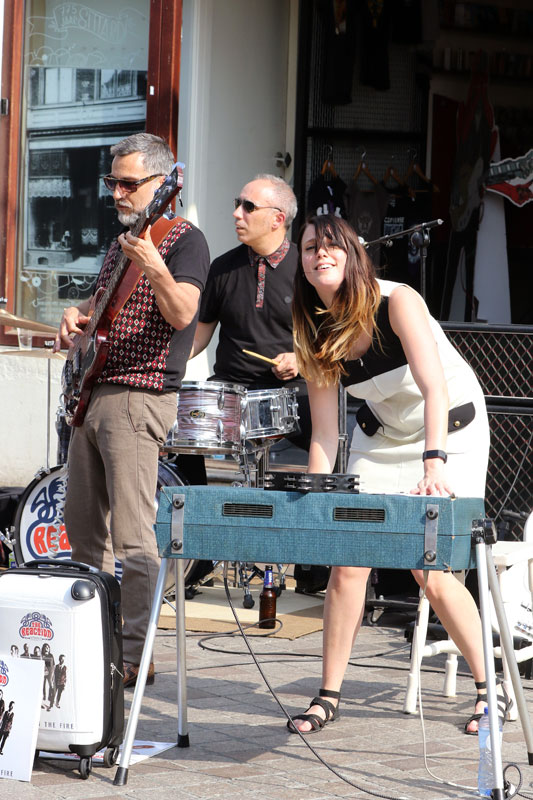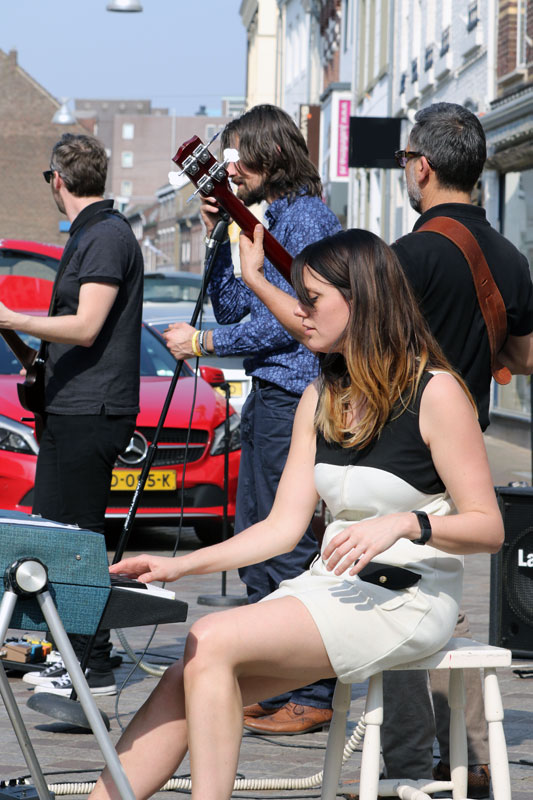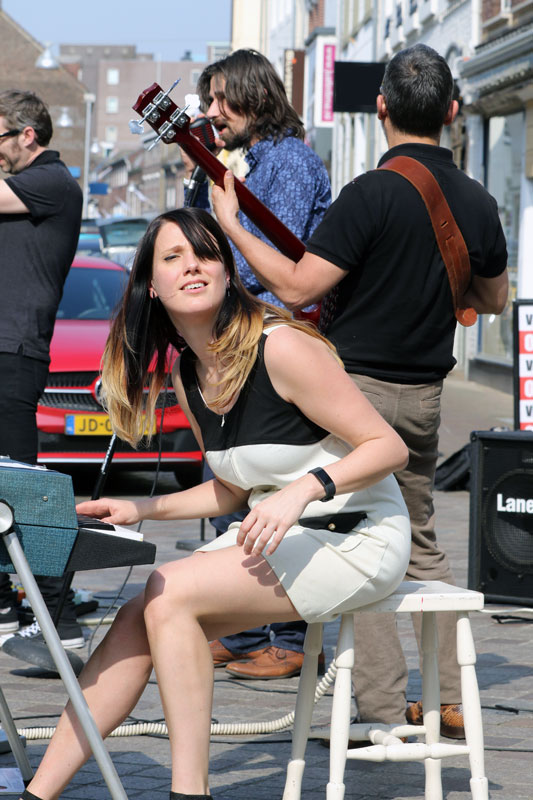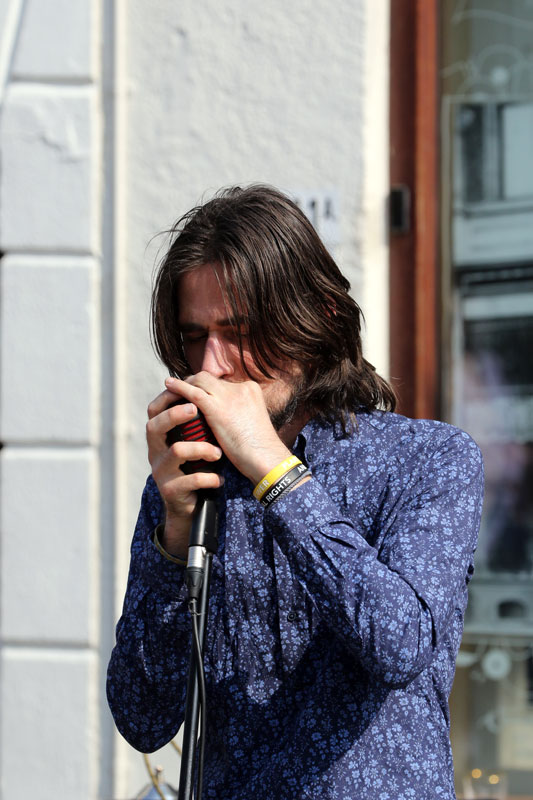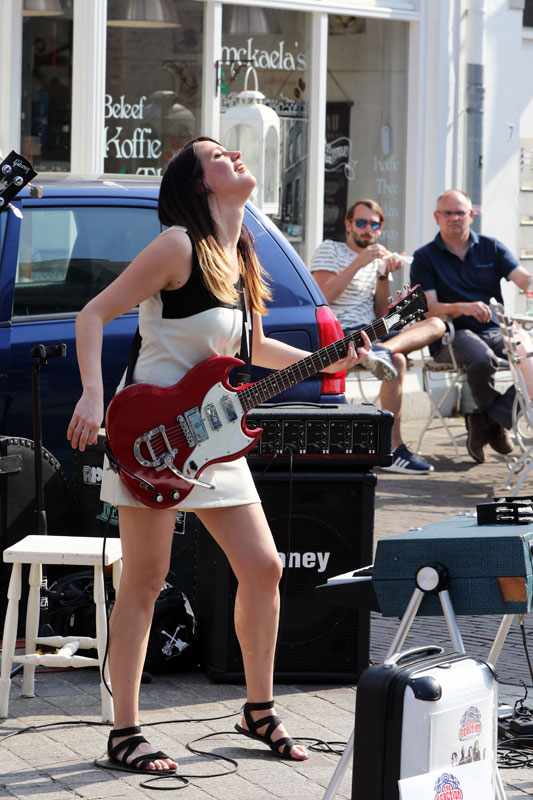Record Store Day, 21 april 2018, Music Machine, Sittard (NL)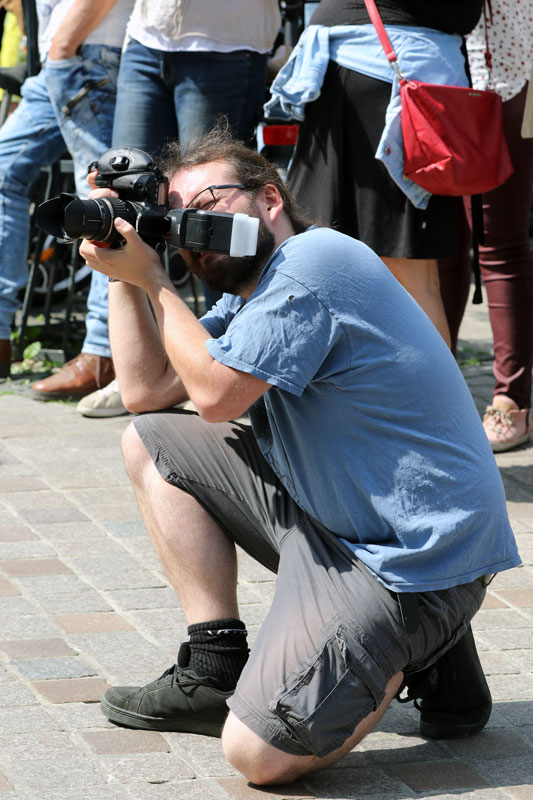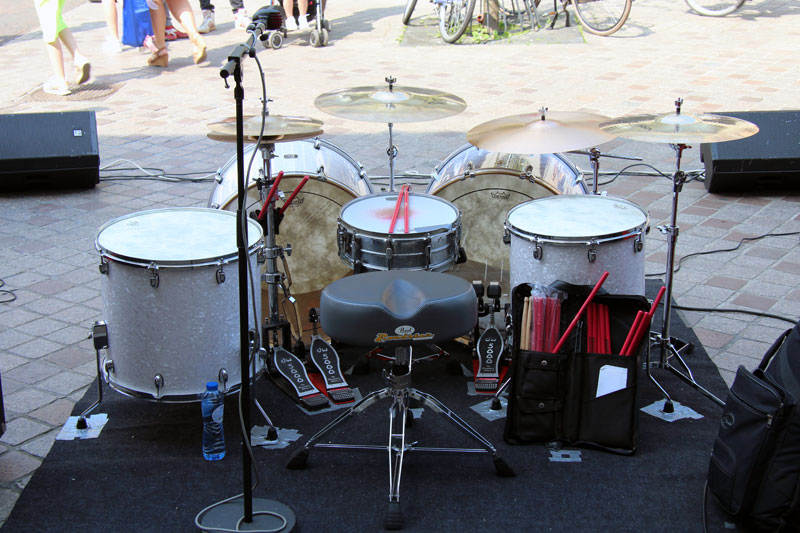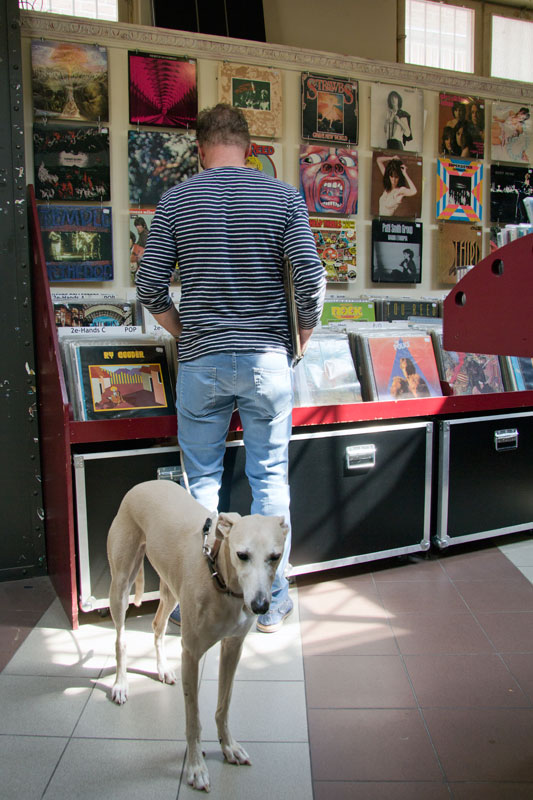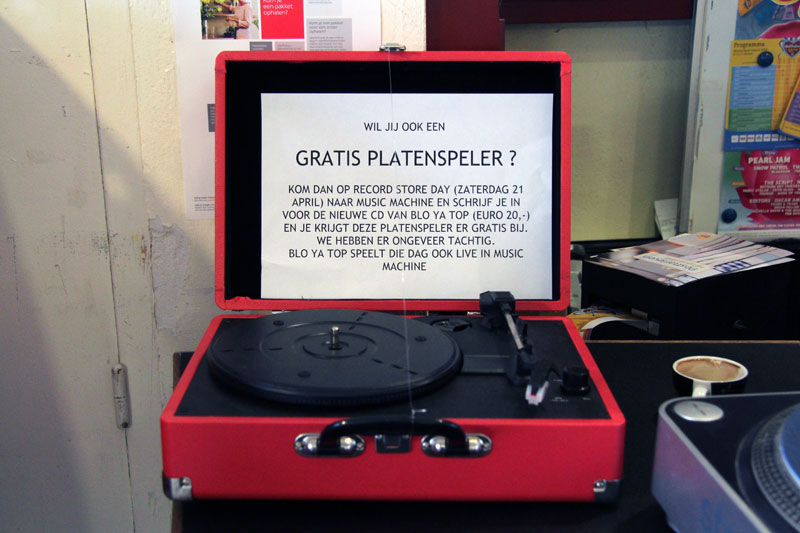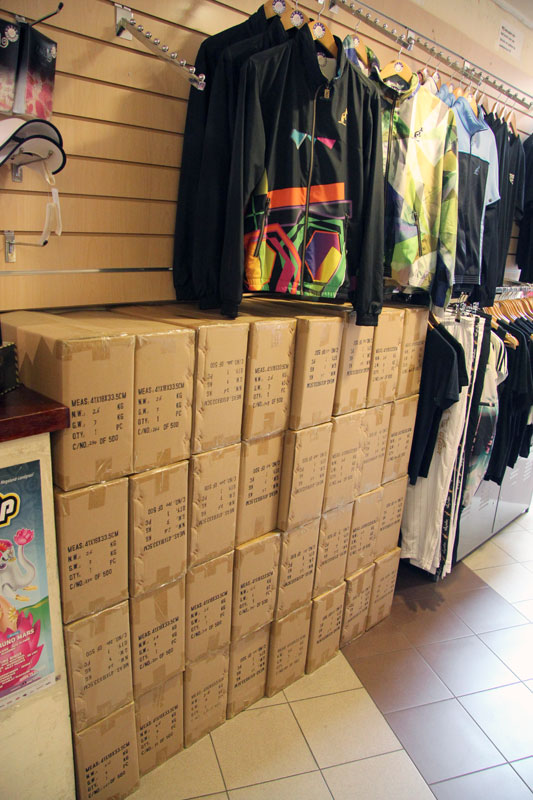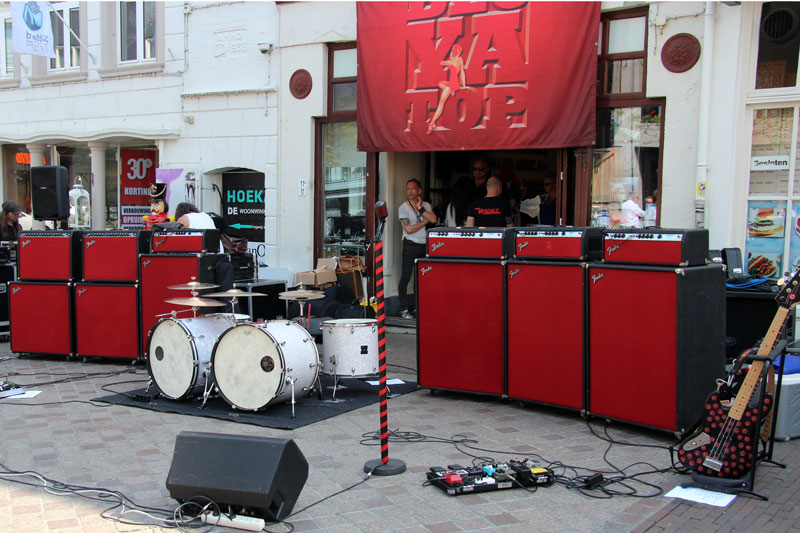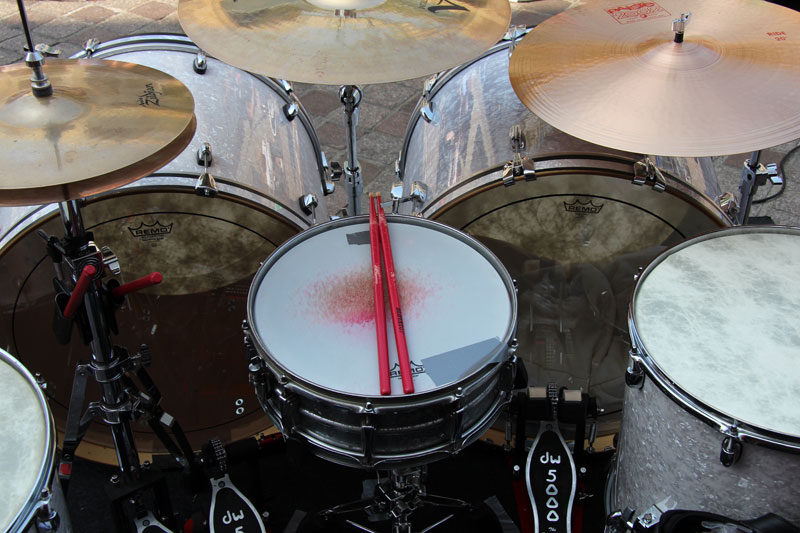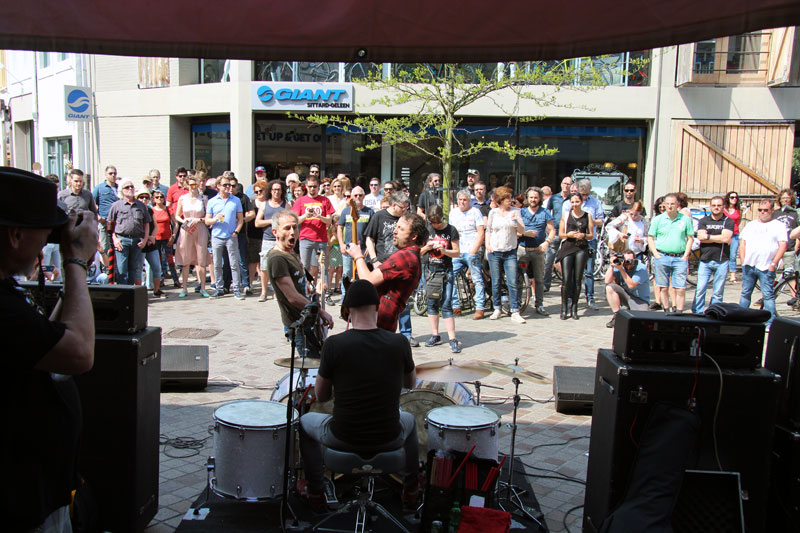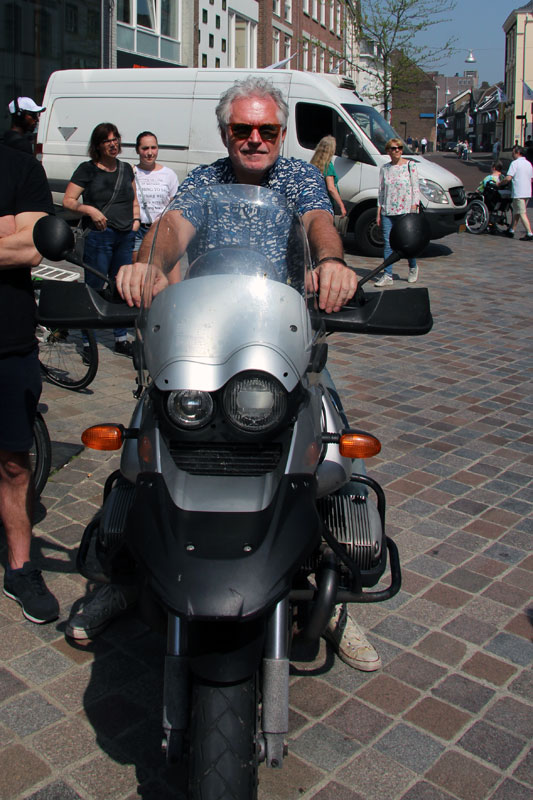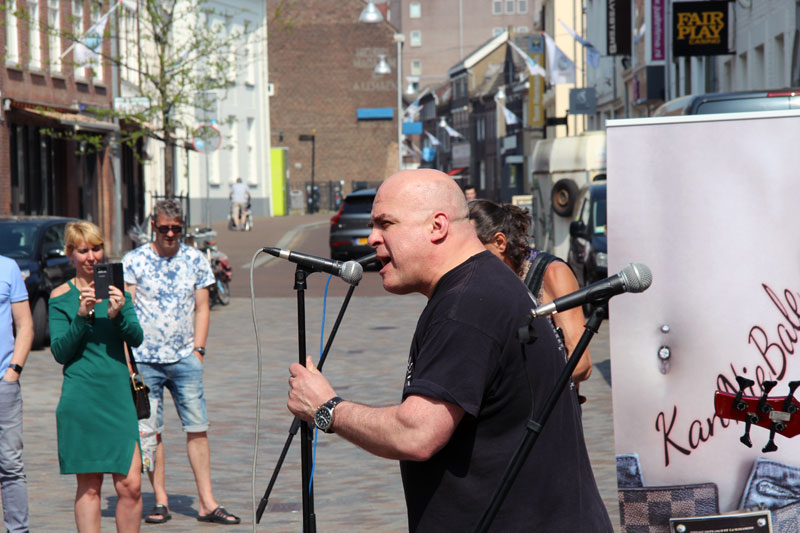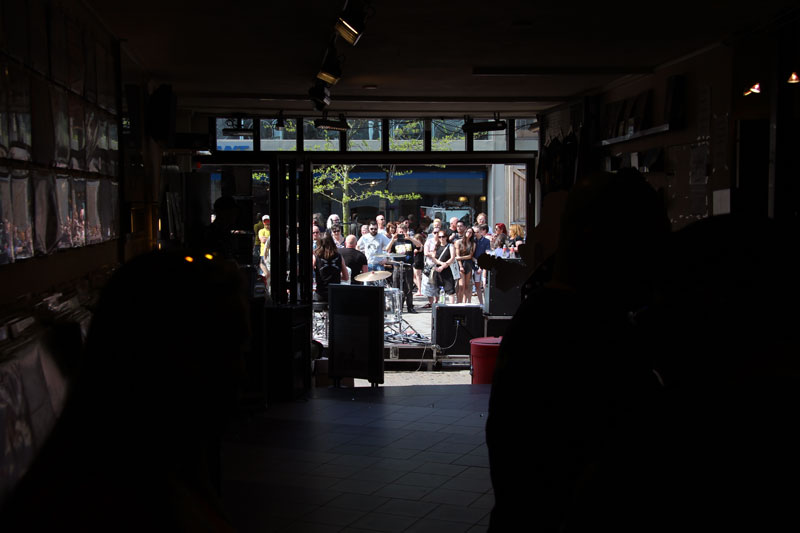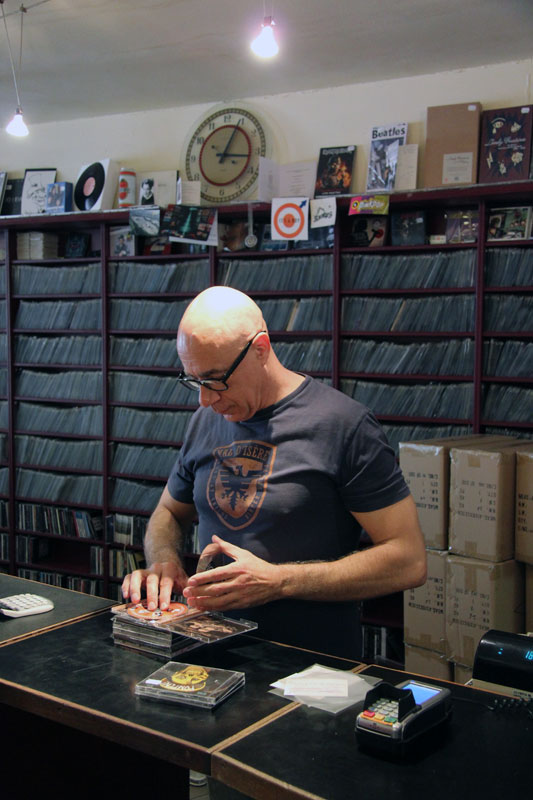For other concert photography click here. C o n c e r t p h o t o g r a p h y C o n c e r t p h o t o g r a p h y C o n c e r t p h o t o g r a p h y C o n c e r t p h o t o g r a p h y C o n c e r t p h o t o g r a p h y C o n c e r t p h o t o g r a p h y C o n c e r t p h o t o g r a p h y C o n c e r t p h o t o g r a p h y C o n c e r t p h o t o g r a p h y C o n c e r t p h o t o g r a p h y C o n c e r t p h o t o g r a p h y C o n c e r t p h o t o g r a p h y C o n c e r t p h o t o g r a p h y C o n c e r t p h o t o g r a p h y C o n c e r t p h o t o g r a p h yThis site created with KompoZer with additional HTML-editing in Kate